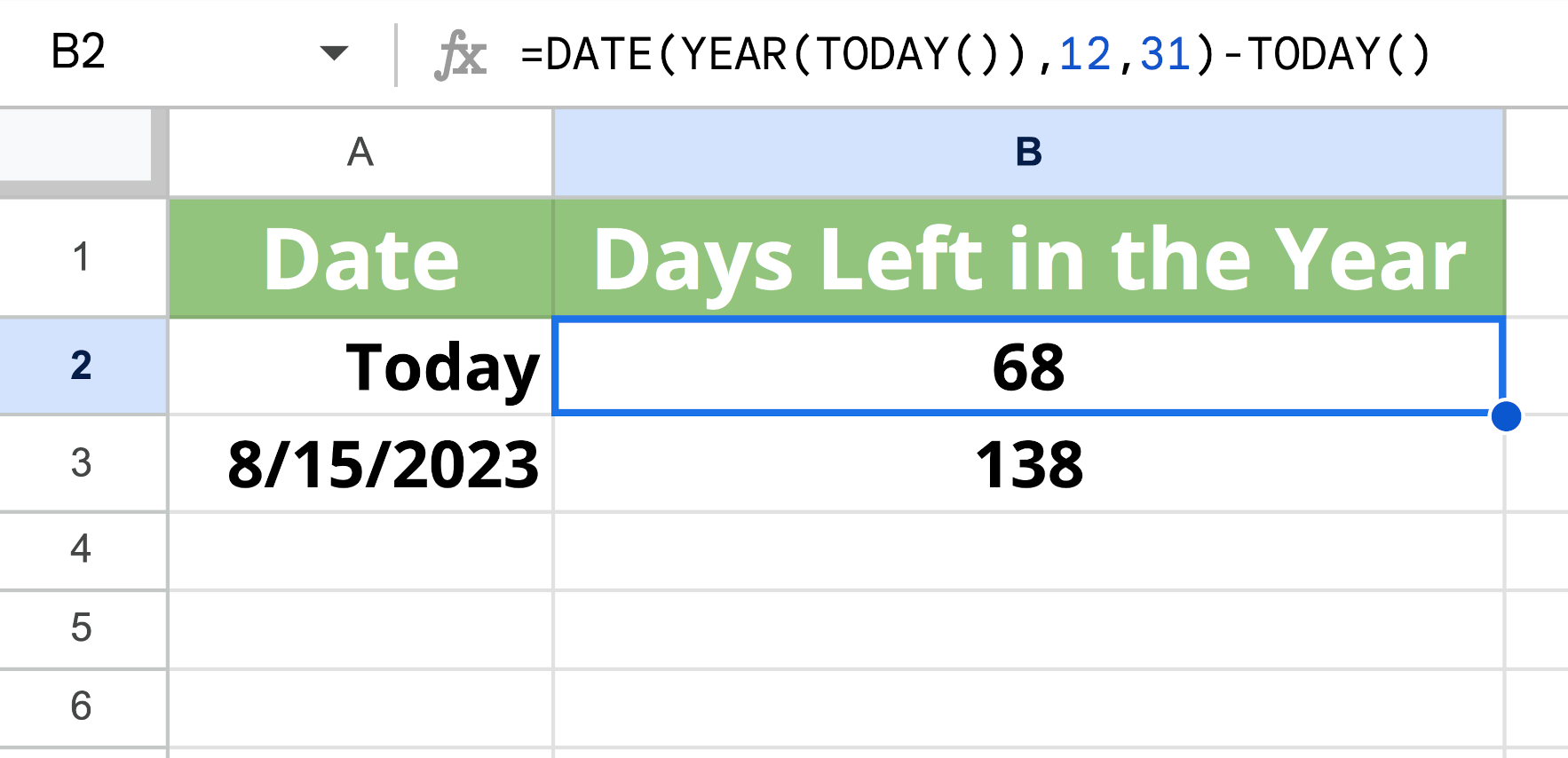# How Many Days Are Left in 2023? Time Left in the Year

Are you trying to figure out how many days are left in the year?29 days

## Time Remaining in the Year:

• 0 months 29 days
• 4 weeks 1 day
• 29 days
• 696 hours

There are twenty-nine days remaining in 2023 from today's date, which is December 2, 2023. You can validate this result using our New Year's countdown calculator.

The following chart shows the days remaining in the year from today and various other days.
Start DateDays Left in the Year
November 28, 202333 days
November 29, 202332 days
November 30, 202331 days
December 1, 202330 days
December 2, 202329 days
December 3, 202328 days
December 4, 202327 days
December 5, 202326 days
December 6, 202325 days

## How To Calculate How Many Days Are Left in 2023

You can count the days left in 2023 in a few ways. The easiest is to use a calculator, such as our days between dates calculator. You can also calculate how many days are left this year manually.

### Method One: Subtract Days in Each Month

If the current date falls in December, then you can simply subtract the current day of the month from 31 since there are 31 days in December. The result is the number of days left in the year.

days left in 2023 = 31 – current day

If the current date is not in December, then you can subtract the current day of the month from the number of days in the month to find the remaining days in the current month. Then, you can add the number of days in each month remaining in the year.

days left in 2023 = days left in current month + days in next month + … + days in December

By dividing this result by 7, you can also calculate how many weeks are left in the year.

You can use the same method to find how many days or weeks are left until Easter, Thanksgiving, or any other significant date.

### Method Two: Subtract the Current Day Number

Another method you can use to count down the days remaining in the year is to subtract the current day number from 365 (or 366 for leap years).

days left in 2023 = 365 – day of the year

To use this method, you need to know the current day of the year.

## How Many Work Days Are Left in the Year?

### Weekdays Left in 2023

21 days

It's important to note that this date does not consider holidays that may fall on a weekday, such as Christmas. So, you'll need to adjust this to account for holidays that you do not work.

## How To Calculate the Remaining Days Using Google Sheets

You can also calculate the number of days remaining in 2023 using spreadsheet software such as Google Sheets or Microsoft Excel. You can do this using a few different formulas.

### From the Current Date

If you want to find the number of days left from the current day, you can use the following function in a cell to display the number of days remaining:

=DATE(YEAR(TODAY()), 12, 31) - TODAY()

For instance, if the current date is October 24th, then the formula might look like this:### From Any Date

If you want to find the number of days left from a date in another cell, for instance, cell A3, then you can use the following function in a cell to display the number of days remaining from the date in cell A3:

=DATE(YEAR(A3), 12, 31) - A3

For instance, if there is a date value in cell A3, then the formula might look like this:You can also use these formulas to calculate the date 60 days, 90 days, or even 120 days from now.